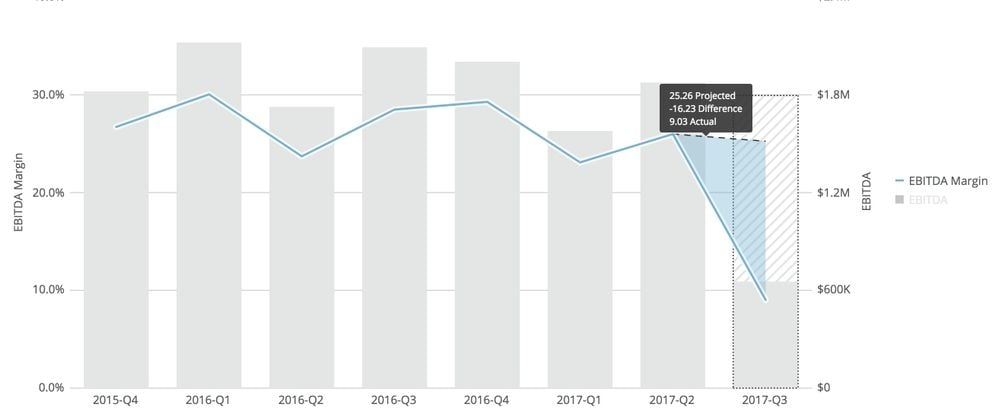# Projection value per category / Plan vs Actual

Hi Domo,

I have the graph attached showing monthly expenses of the company in a form of stacked bar. When I add projection value it is same for each expense category. Is it possible to stated separate projection value to each category within last month?

In case it is not possible, I want at least total per month to be projected: stacked bar with the total projected per month. Or is it possible to add the forecast (budget) line showing total planned expenses per month and how much "budget" left for the last month

• I see.  Thanks for the clarification.

You'll have to bring that projection Excel data into a dataset with your actuals.  You'd stack them together in such a way that the projection amount goes in the same column as your actual amount.  Likewise, your projection expense type would go in the same column as actual expense types software, advertising, etc.  You're almost there with that second chart.

Then to get the projections to appear as the line, you'll probably need to sort the data with a beast mode to force the ordering of expense types. That calculation would look something like:

CASE WHEN `type` = 'Projection' THEN 1 WHEN `type = 'Software' THEN 2 .... END

Just make the projection type first, then you can make the rest go in any order you want.

• I think what you're looking for is a different kind of projection, right?  You've chosen Specified Value, which will give each series the same amount. That's probably not at all helpful.  Expense categories don't rise or fall in lock step

Chose a different projection method in that dropdown, like Linear Regression, to give each series its own calculated value.  Each expense category will have its own growth pattern here.

• Thanks. But linear regression will calculate the projected amounts which will be different from what I have. We have calculated projected amounts in excel file and now I just want to illustrate our actuals compared to projected amounts.

I will be also good if the projected amount will be total for all expenses I have. So we could see it in the following wayI also tried to use line + stacked bar. I wanted to use line as projected total expenses (not sure also how the chart selects what category should be line and what shoul be bars) but there is no "last value projection" option within chart properties in line + stacked bar, thus I can't see how far my actuals prom projection are• Thanks, that looks good enough.

The last thing I'd like to figure out is if it possible to have the actual to projection comparison like it is in one of the Demo charts (last value projection used here). Unfortunately, I don't see "last value projection" in the line + stacked bar chartThis discussion has been closed.